# Working with sigmoid in Solidity## Introduction

While working on a project in solidity, I needed to make a calculation with Sigmoid. Now, anyone who has already worked with sigmoid will know that it involves the irrational number e. In this article, I will describe what problems I faced and how I tried to approach the problem from a mathematical and programming concept.

## The problem

Solidity does not have native support for fractional operations, instead, it uses a scaled version of numbers (i.e: uint256 and int256) to make operations for fractional calculation. for example, if you are to do an operation like this:

``let x = 1/3;``

In pure solidity the result needs to be scaled to adjust precision similar to this:

``uint256 x = (10**18)/3;``

On top of that, the natural exponential function makes it harder to calculate in pure solidity.
## Solution Approach

I tried to solve this problem using observation and estimations using the graph. Let's look at the graph for the sigmoid function: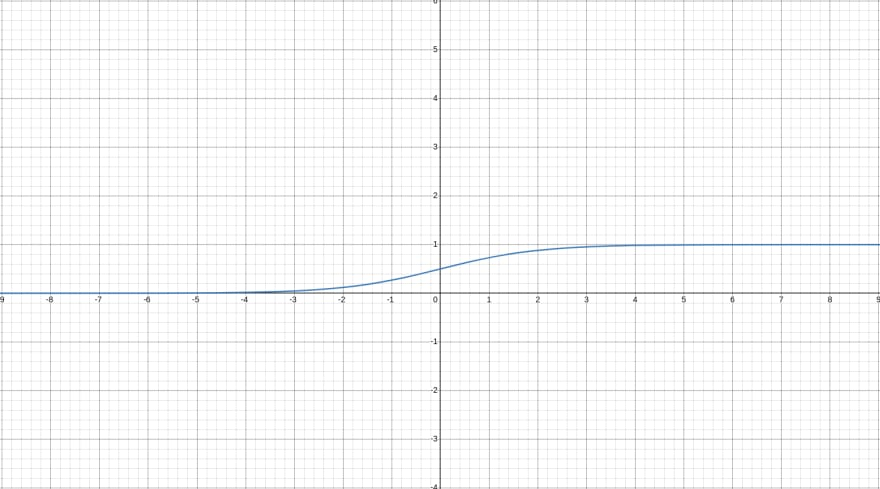As x approaches 5 and goes to infinity, the function result tends to reach 1. And as x approaches -5 and goes to -infinity, the function results in 0.

The first observation that I got from this is that the graph is symmetric for positive and negative x. If we shift the graph by 0.5 units below the y-axis, we will see that x and -x yield the same result but in opposite directions on the y-axis.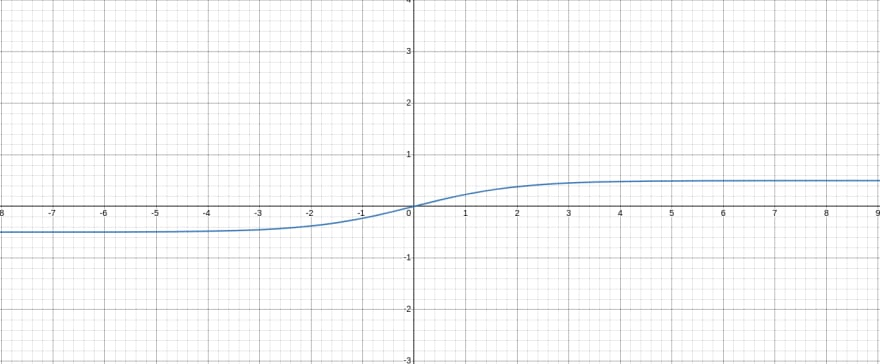So, we can calculate the values for negative x by adding/subtracting some offset value with the positive x value. From the shifted graph, we can calculate the value for this offset. We shifted the y values by 0.5, and we got relation for positive and negative x like this:
From this equation we get: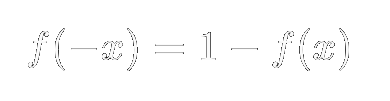We can now use the positive x to calculate the values for negative x.

## Polynomial estimations

We have reduced the problem domain in half, but the irrational number and the fractional calculations are still not solved. What if we could express the function in some simpler polynomial function? We could use the Maclaurin series to achieve this. Taking the first 6 terms for the Maclaurin expansion for the sigmoid function, we get: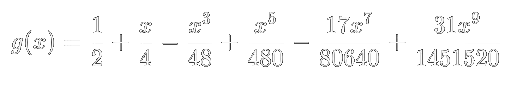If we plot g(x) and f(x) in the graph, we will see something like this: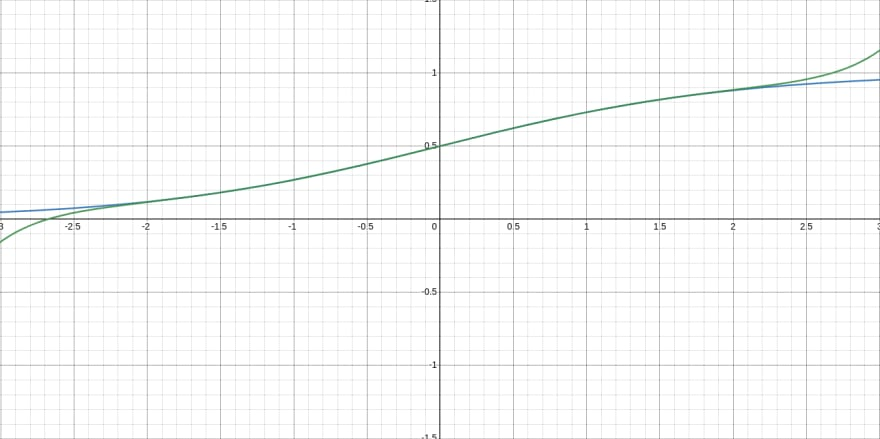The green curve is g(x) and the blue curve is f(x). We can see that the estimation does very well from 0 to 2 but diverges from the original curve after that. So, we can use this for values from -2 to 2 in our code.

## Linear estimations

After that, I used linear estimations for the rest of the values. I found that from 2 to 5 I needed a linear estimation and from 5 to 9 to smooth out the results. Anything greater than 9 or less than -9, I assumed to be 1 and 0 respectively.

## Polinomial estimation code

To implement the curve in solidity in simple terms, I processed the mathematical term a bit. It made it easier to write the solidity code. The denominator of the term was 1451520. I also calculated the modifiers for the x nominators.

``````function buildModifiers() internal pure returns (uint256[] memory) {
uint256[] memory modifiers = new uint256[](5);
modifiers = uint256(362880);
modifiers = uint256(30240);
modifiers = uint256(3024);
modifiers = uint256(306);
modifiers = uint256(31);
return modifiers;
}``````

As I have scaled the numbers by 10^18 I used a power function to scale down. One observation from the polynomial term is that all the power of x is odd, and thus the next x term can be obtained by multiplying the previous x term with x^2. I incorporated that into my code as well.

``````function power(uint256 base, uint256 scale)
internal
pure
returns (uint256[] memory)
{
uint256 square = (base * base) / scale;
uint256[] memory result = new uint256[](5);
result = base;
for (uint i = 1; i < 5; i++) {
result[i] = (result[i - 1] * square) / scale;
}
return result;
}``````

Lastly, I multiplied the result with the modifiers and add/subtract the terms to get the answer.

``````function polynomial(
uint256 base,
uint256 scale,
uint256[] memory modifiers,
) internal pure returns (uint256) {
uint256 result = 0;
uint256[] memory powers = power(base, scale);
for (uint i = 0; i < 5; i += 2) {
result += modifiers[i] * powers[i];
}
for (uint i = 1; i < 5; i += 2) {
result -= modifiers[i] * powers[i];
}
return result / denominator + addition;
}``````

The addition variable is the value for f(0) which is 0.5 scaled by 10^18.

## The result

As I used fixed polynomials and linear approximations to calculate the sigmoid, I was able to calculate the result in constant time. So, the time complexity for this function was O(k) or O(1). And with 10000 different inputs for sigmoid, the final result had s mean absolue error of 0.0001319 or 0.01319%

## Conclusion

I had a lot of fun working on this particular problem. It was exciting to use high school math along with observations to implement something that is natively not available in solidity. I wish to do more exciting stuff like this in the future as well. Happy coding everyone!